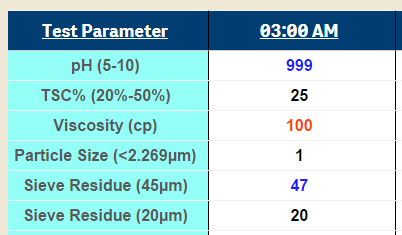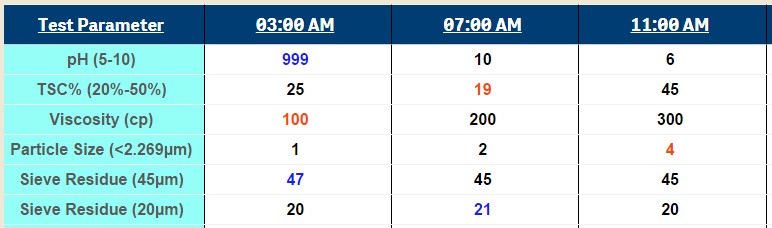New to Qlik Sense

Discussion board where members can get started with Qlik Sense.

Announcements
QlikWorld 2020: Join us May 11 - 14, 2020 in Phoenix, AZ. Register early and save \$400. Learn More
Highlighted
New Contributor III

how to calculate row by row with different calculation

Hi master. is there any way to have a different calculation by row? lets say that (999 * 25) + 100 -1 +(47/20). the issue now i had problem to point that 999, 25, and the rest since use 2 dimension. kindly to have your help. tq in advance.Tags (2)
5 Replies
Contributor III

Re: how to calculate row by row with different calculation

We can write different calculation for each row by using the Dimension value hard coded or using the Rowno() function.

For example Using Rowno():

If (Rowno()=1, 100+50,

If (Rowno()=2, 100-50))

For example Using Dimension value hardcoded:

if(Parameter ='pH', 100+50,

if(Parameter ='Viscosity', 100-50))

Not sure I fully understand what you exactly want to get, Please sharesample application.

Thanks,

Sibin

New Contributor III

Re: how to calculate row by row with different calculation

thanks for your time sibin.

actually, my client have requirement to calculate those columns into 1 value.

lets say an example.

total for 3AM= (999 * 25) + 100 -1 + (47/20)

total for 7AM= (10*19) + 200 - 2 + (45/21)

and the rest follow the same rules.

actually idk whether i can share here since i use extension. hahaContributor III

Re: how to calculate row by row with different calculation

Hi,

I am assuming,the straight table having one dimension (Test Parameter) and 3 expressions.

and you need a specific calculation for Total column in "3AM" and "7AM"

Go to expression tab , Total Mode -> select "Expression Total" option.

then change expression definition of 3AM as below

if(isnull(RowNo()),(999 * 25) + 100 -1 + (47/20),

existing expression)

expression definition of 7AM

if(isnull(RowNo()),(10*19) + 200 - 2 + (45/21),

existing expression)

Thanks,

Sibin

Contributor III

Re: how to calculate row by row with different calculation

Hi,

Correct if i understand you question wrong,

This is your formula: (999 * 25) + 100 -1 + (47/20)

You can write a expression like:

Expression 03:00 AM:

(Sum({<TestParameter={'pH(5-10)'}>}03:00 AM) * Sum({<TestParameter={'TSC % (20% - 50%)'}>}03:00 AM) ) + Sum({<TestParameter={'viscosity(cp)'}>}03:00 AM)-Sum({<TestParameter={'Particle Size(2.269um)'}>}03:00 AM)+(Sum({<TestParameter={'Seive Residue (45um)'}>}03:00 AM) / Sum({<TestParameter={'Seive Residue (20um)'}>}03:00 AM))

Expression 07:00 AM:

(Sum({<TestParameter={'pH(5-10)'}>}07:00 AM) * Sum({<TestParameter={'TSC % (20% - 50%)'}>}07:00 AM) ) + Sum({<TestParameter={'viscosity(cp)'}>}07:00 AM)-Sum({<TestParameter={'Particle Size(2.269um)'}>}07:00 AM)+(Sum({<TestParameter={'Seive Residue (45um)'}>}07:00 AM) / Sum({<TestParameter={'Seive Residue (20um)'}>}07:00 AM))

Expression 11:00 AM:

(Sum({<TestParameter={'pH(5-10)'}>}11:00 AM) * Sum({<TestParameter={'TSC % (20% - 50%)'}>}11:00 AM) ) + Sum({<TestParameter={'viscosity(cp)'}>}11:00 AM)-Sum({<TestParameter={'Particle Size(2.269um)'}>}11:00 AM)+(Sum({<TestParameter={'Seive Residue (45um)'}>}11:00 AM) / Sum({<TestParameter={'Seive Residue (20um)'}>}11:00 AM))

Thanks,

New Contributor III

Re: how to calculate row by row with different calculation

thanks for ur help sir.

unfortunately my client ask me another way around.

lets say total is been calculated by within range (black colour).

they want :

total for 3AM= (3(black) / (6(All colour)) *100 = 50%.

total for 7AM= (4(black) / (6(All colour)) *100 = 66.6%.

is it possible? maybe u can share other mechanism instead of color

i share the query for color.

if([Test Parameter] like 'pH (5-10)' AND [03:00AM]>=5 AND [03:00AM]<=10,BLACK(),

if([Test Parameter] like 'TSC% (20%-50%)' AND [03:00AM]>=20 AND [03:00AM]<=50,BLACK(),

if([Test Parameter] like 'Viscosity (cp)' AND [03:00AM]>=200 AND [03:00AM]<=500,BLACK(),

if([Test Parameter] like 'Particle Size (<2.269µm)' AND [03:00AM]<3,BLACK(),

if([Test Parameter] like 'Sieve Residue (45µm)' AND [03:00AM]=45,BLACK(),

if([Test Parameter] like 'Sieve Residue (20µm)' AND [03:00AM]=20,BLACK(),rgb(256,256,256))